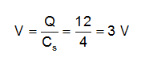# Calculate the capacitance of 6uF and 12uF are connected in series

Calculate the capacitance of 6uF and 12uF are connected in series with a battery. The voltage across the 6uF capacitor is 2V, compute total battery voltage.

The series capacitance is given as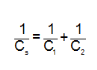Thus, the total resistance across the circuit is;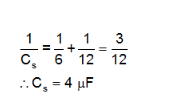The charge across a capacitor is
?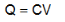Thus, charge across 6 ?F capacitor is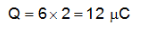Since, the combination is a series combination, the charge remains the same across entire circuit.
Thus, the total voltage of the battery is